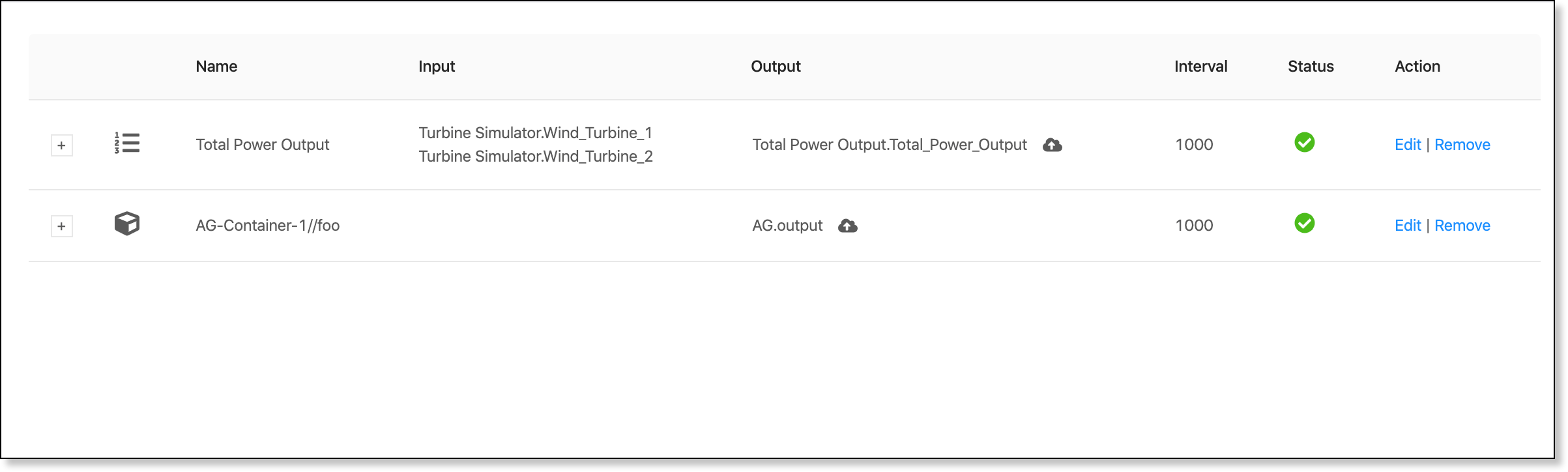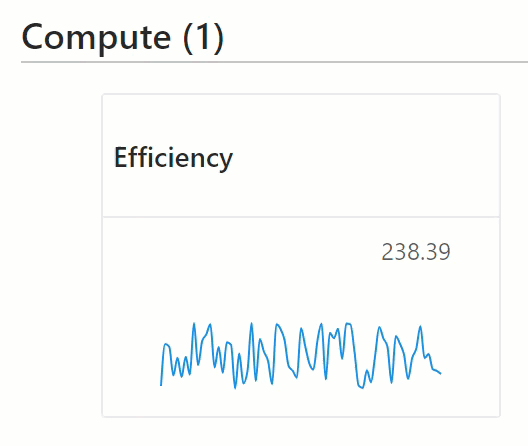Compute Blocks¶

A compute block is a mathematical function that derives its value from an expression. Compute blocks give you the ability to calculate new tag values based on historical tag data collected from interfaces, models, or even other compute blocks.

Example

In the example below, the Total Wind Farm Output compute block calculates one output (Total_Power_Output) using two inputs (Wind_Turbine_1 and Wind_Turbine_2).You can use a compute block to calculate one or multiple output tags.

Guidelines¶

Review the following guidelines when building compute blocks.

Update Criteria¶

The Update Criteria for a compute block determines when the value of the output tag changes based on new values for its input tags.

Following are the options available:

All Inputs Changed

The compute block will produce an output if all input tags change value.

For example, if a compute block has two input tags, A and B, and their last observed values are 2 and 3, respectively. The compute block will produce an output if A changes to 5 and B changes to 4. If only A changes to 5, the compute block won't produce a new output until B also changes.

Any Input Changed

The compute block will produce an output if any input tag changes value.

For example, if a compute block has two input tags, A and B. The compute block will produce an output if A and/or B change value, including if both A and B change at the same time.

The compute block will produce an output if all input tags receive a new value.

For example, if a compute block has two input tags, A and B, and their last observed values are 2 and 3, respectively. The compute block will produce an output if A receives a new value of 2 and B receives a new value of 4.

The compute block will produce an output if any input tag receives a new value.

For example, if a compute block has two input tags, A and B. The compute block will produce an output if A or B receive a new value. The compute block will also produce an output if both A and B receive a new value at the same time.

Historical Tag Values¶

You can access up to 20 historical values for an input tag and use these values in the expression for a compute block.

To specify the historical values to use for an input tag, select an option in the History picklist for that tag.

Example

• Select "Last value only" in History to use the latest value
• Select "20 element arrays" in History to use the last 20 values

To insert specific input tag values into an expression, enter the tag ID followed by an index [x]. For example, the following expression calculates the value of the Difference tag by subtracting the two latest values for the TotalLength tag.

 1 Difference = TotalLength.values - TotalLength.values

Along with a tag's value, you can also insert the following input tag attributes into an expression:

• .timestamps
• .epochtimes
• .qualities
• .lengths

Expressions¶

An expression calculates the output tag(s) for a compute block. To build an expression, you need to include the input tags for the compute block along with mathematical operators and functions.

Info

Click the i icon to view more information about defining expressions.

Here is an example of an expression that calculates the efficiency for a pump.

 1 2 3 4 gacc = 9.80665 p_diff_m = p_diff * 100.0 / gacc sg(temperature) = (999.65 + 0.20438 * temperature - 0.061744 * temperature ^ 1.5) / 1000.0 efficiency = (flow_rate * p_diff_m * sg(temp) * gacc / (power * 3600.0 * 0.906)) * 100

The input tags are:

• power
• flow_rate
• p_diff
• temp

The output tag is:

• efficiency

Notice how the gacc, p_diff_m, and sg(temperature) values are calculated first, and then inserted into the efficiency calculation (line 4) that determines the value for the output tag.

Building a Compute Block¶

Complete the following to build a new compute block.

1. Select Compute Chain on the sidebar.
2. Click Add Compute Block.
3. In Name, enter a name for the compute block.
4. In Update Criteria, specify when you want the output tag to change based on its input tags.

• See the Guidelines section above for more information about update criteria.
5. (Optional) In Description, enter a description of the output value of the compute block.

6. In Interval, specify the interval in milliseconds at which to update the output value.
7. Under Inputs, complete the following for every input tag:

1. Select the tag to use:

• Use a tag from an interface: Select the data source in Interface and select the tag in Tag ID.
• Use an output tag from a compute block: Select Compute in Interface and select the output tag in Tag ID.
2. In Expression variable name, enter the local name to use for the tag.

3. Click + Add.
4. Click Edit next to the tag.
5. In History, select the value to use for the tag.

• See the Guidelines section above for more information about historical tag values.
6. Click Save.

8. Under Outputs, complete the following for every output tag:

1. In Output Tag ID, enter a name for the tag.

• This is the name of the tag used to identify the tag in Arundo Fabric.
2. In Expression Variable, enter a local name to use for the tag.

• This is the name of the tag used to identify the tag in the expression for this compute block.
3. Click + Create.

9. In Define the expression, enter an expression to calculate the output tag(s).

• Include input tags in the expression by entering their Expression Variable.
• A built-in syntax checker displays alerts when you enter expression elements incorrectly.
• Visit the math.js site for more information on correct expression syntax.
• See the Guidelines section above for more information about expressions.
10. Click Save.

Viewing Compute Block Output¶

Complete the following to view the calculated outputs for compute blocks.

1. Select Viewer on the sidebar.
2. Open the Graph tab.
3. Move down to the Compute section. Graphs showing the output tags for compute blocks now display.Managing Compute Blocks¶

Complete the following to manage existing compute blocks.

Editing a Compute Block
1. Select Compute Chain on the sidebar.
2. Locate the compute block in the list.
3. Click Edit next to the compute block.
4. Make changes as necessary.
5. Click Update.
Editing an Input Tag
1. Select Compute Chain on the sidebar.
2. Locate the compute block in the list.
3. Click Edit next to the compute block.
4. Under Outputs, click Edit next to the tag.
5. Make changes as necessary.
6. Click Save.
7. Click Update.
Editing an Output Tag
1. Select Compute Chain on the sidebar.
2. Locate the compute block in the list.
3. Click Edit next to the compute block.
4. Under Outputs, click Edit next to the tag.
5. Make changes as necessary.
6. Click Save.
7. Click Update.
Preventing an Output Tag from Streaming to Arundo Fabric
1. Select Compute Chain on the sidebar.
2. Locate the compute block in the list.
3. Click Edit next to the compute block.
4. Under Outputs, click Edit next to the tag.
5. Deselect the checkbox on the left side of the page.
6. Click Save.
7. Click Update.
Deleting an Input Tag
1. Select Compute Chain on the sidebar.
2. Locate the compute block in the list.
3. Click Edit next to the compute block.
4. Under Inputs, click Delete next to the tag.
5. Click Update.
Deleting an Output Tag
1. Select Compute Chain on the sidebar.
2. Locate the compute block in the list.
3. Click Edit next to the compute block.
4. Under Outputs, click Delete next to the tag.
5. Click Update.
Deleting a Compute Block
1. Select Compute Chain on the sidebar.
2. Locate the compute block in the list.
3. Click Delete next to the compute block.
4. Click Delete to confirm.